# RD Sharma Solutions for Class 6 Maths Chapter 5: Negative Numbers and Integers Exercise 5.4

## RD Sharma Solutions for Class 6 Maths Exercise 5.4 PDF

RD Sharma Solutions are designed with the aim of providing better conceptual knowledge among students. Each problem contains step wise solutions in an interactive manner in order to make the subject interesting for students to learn. The students can make use of solutions which are designed by subject experts in order to score well in the Class 6 exam. The solutions are prepared with the aim of helping students self analyse their areas of weaknesses. RD Sharma Solutions for Class 6 Maths Chapter 5 Negative Numbers and Integers Exercise 5.4 are provided here.

## RD Sharma Solutions for Class 6 Maths Chapter 5: Negative Numbers and Integers Exercise 5.4 Download PDF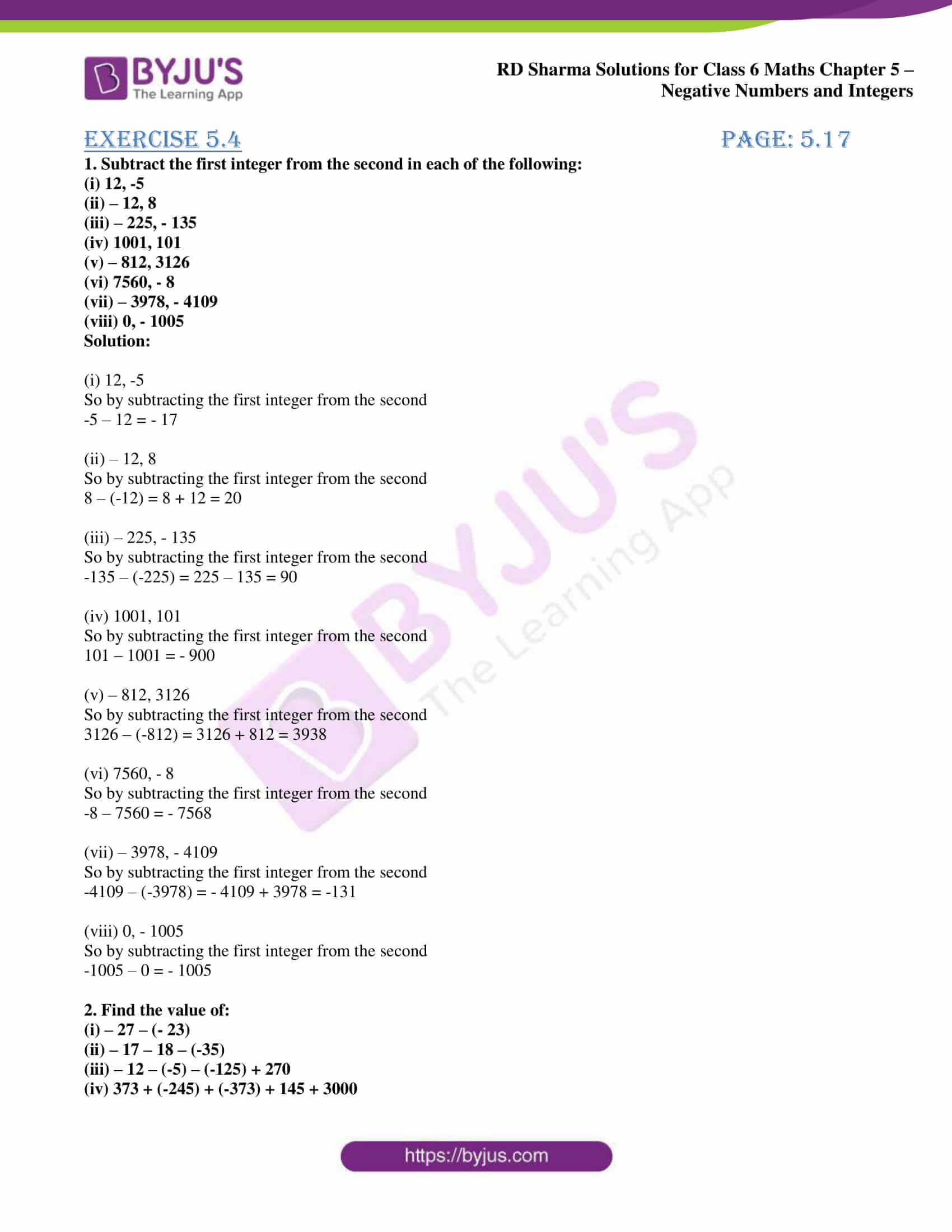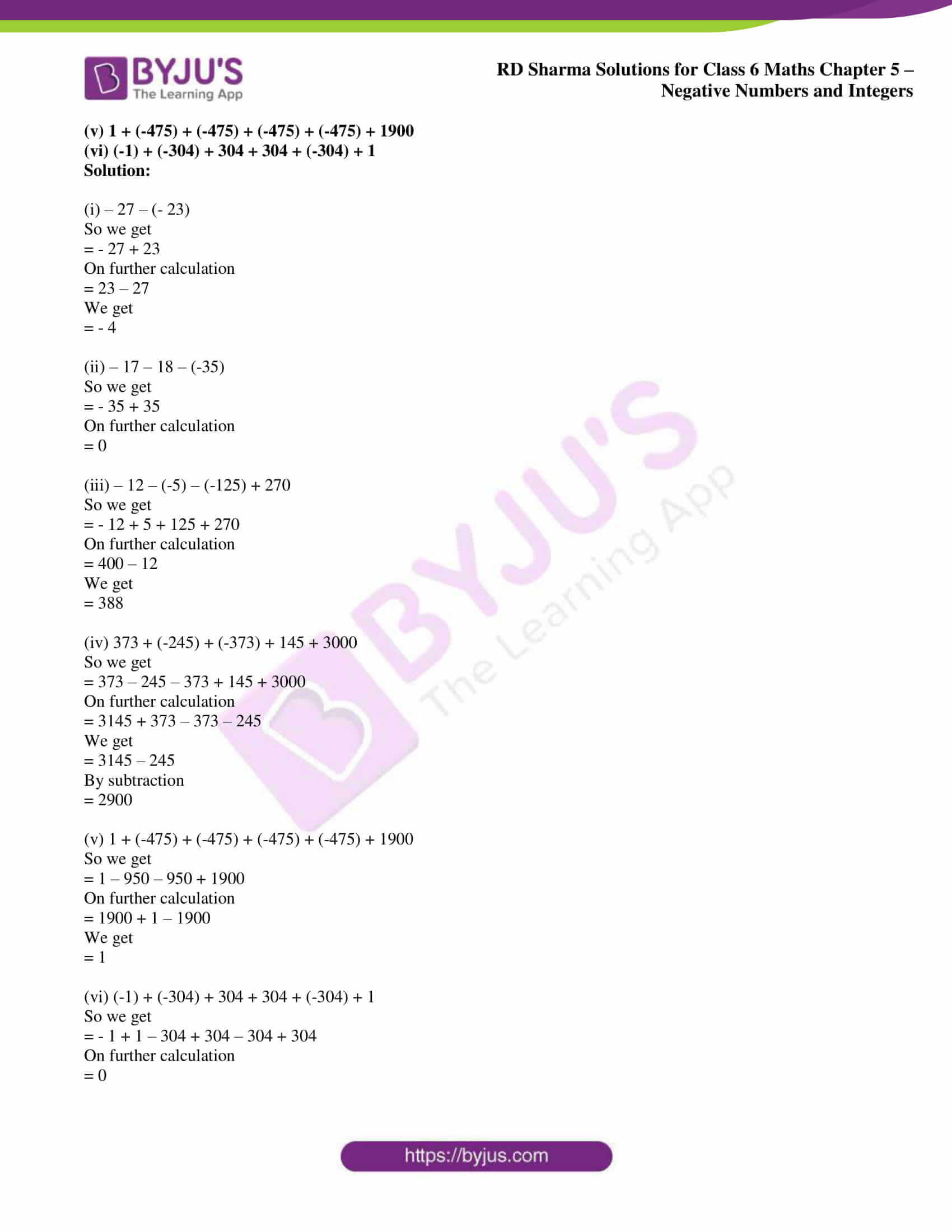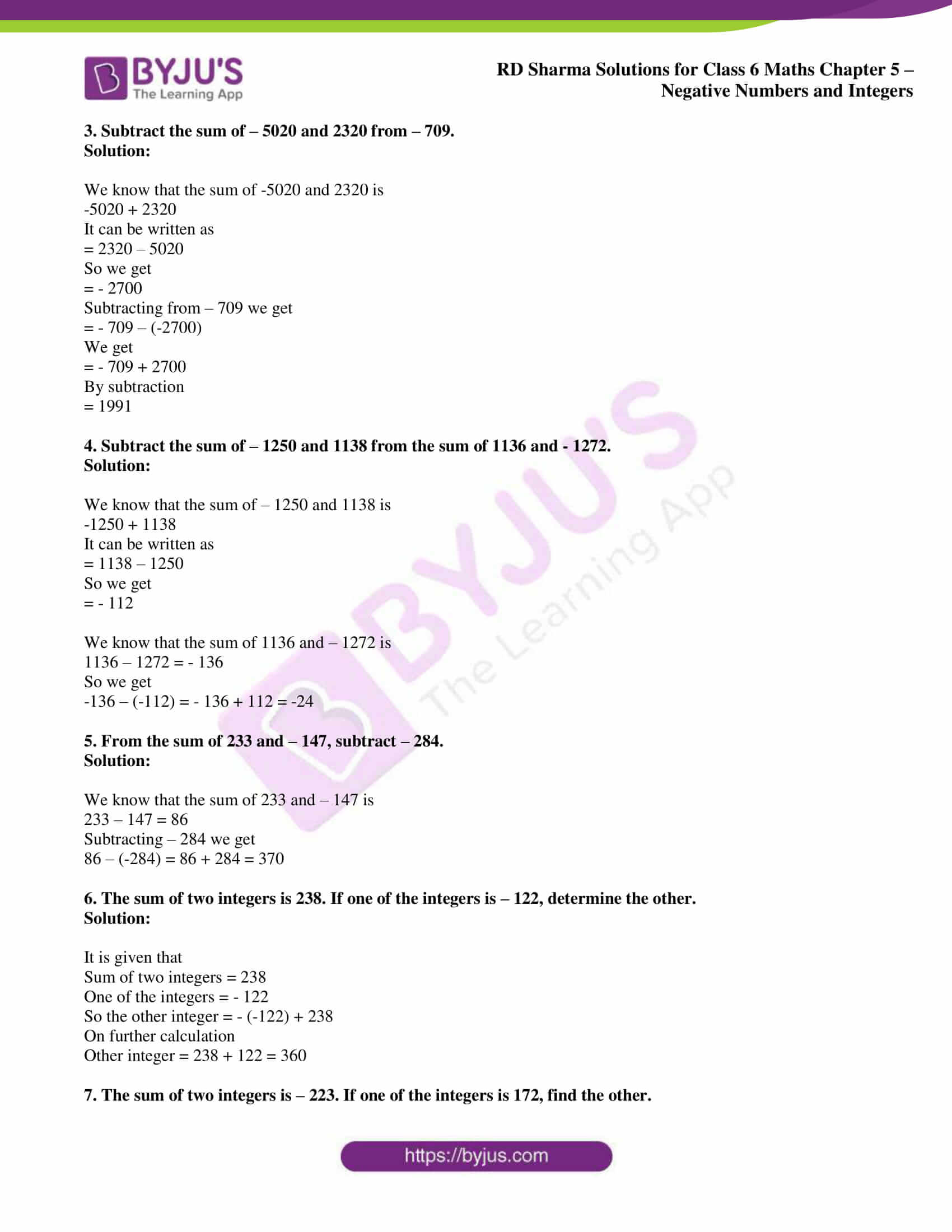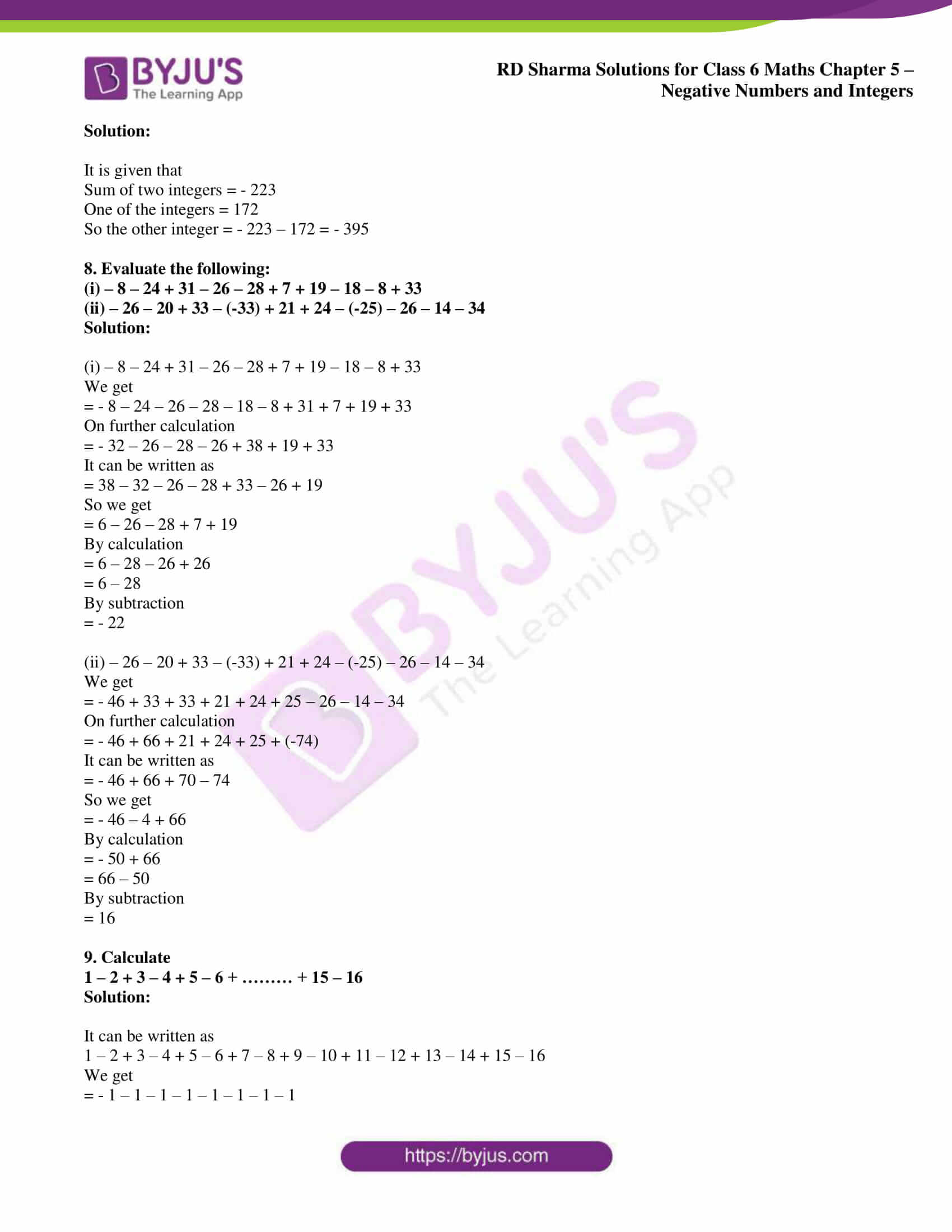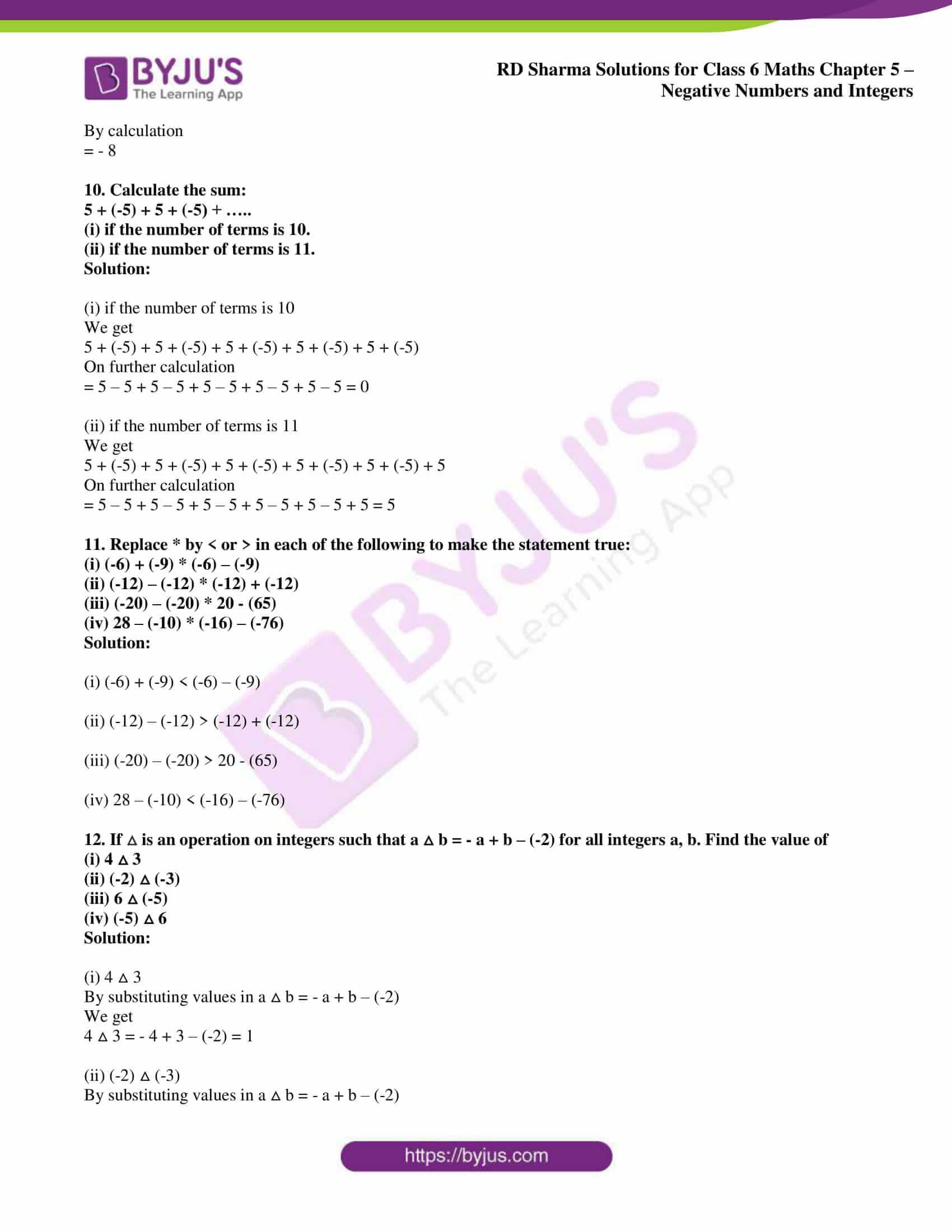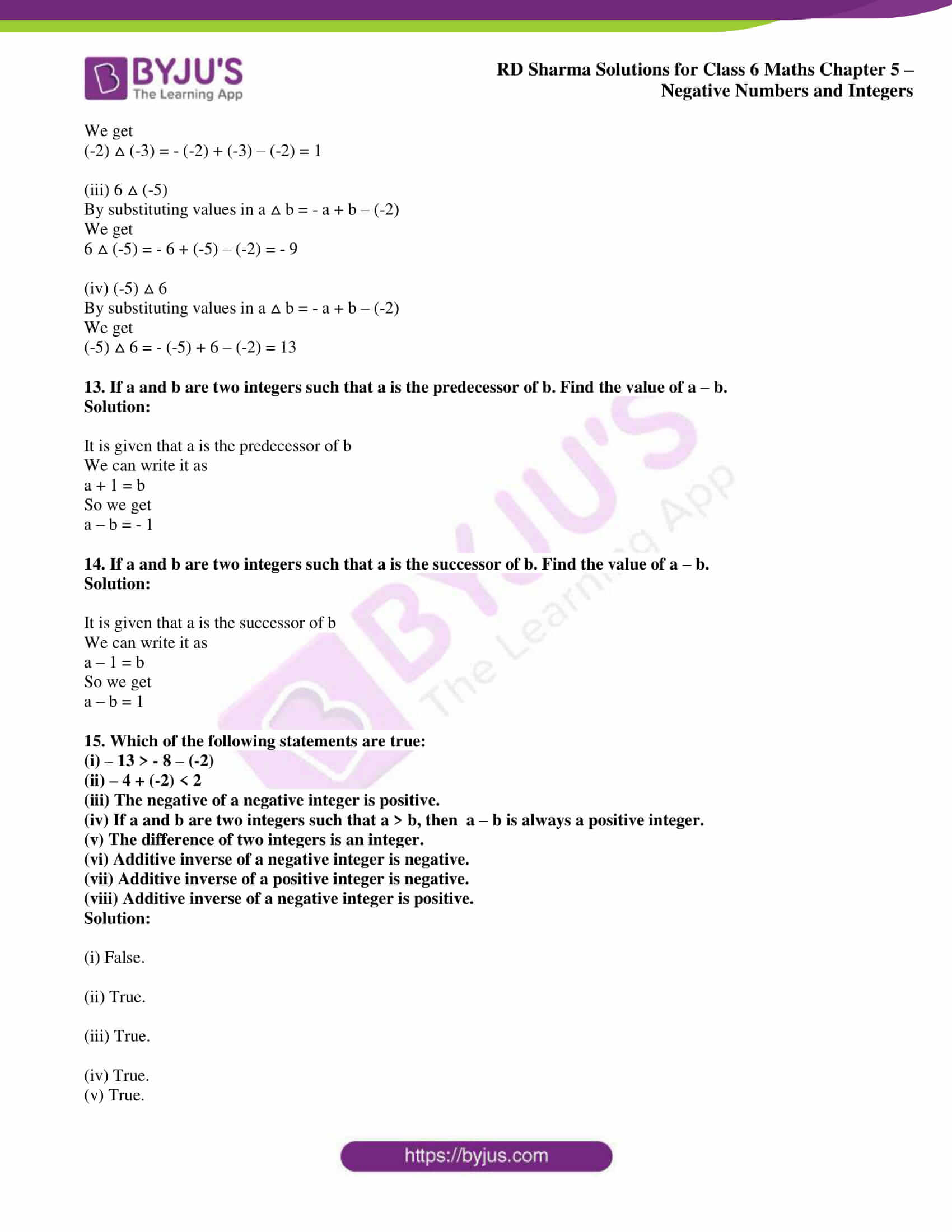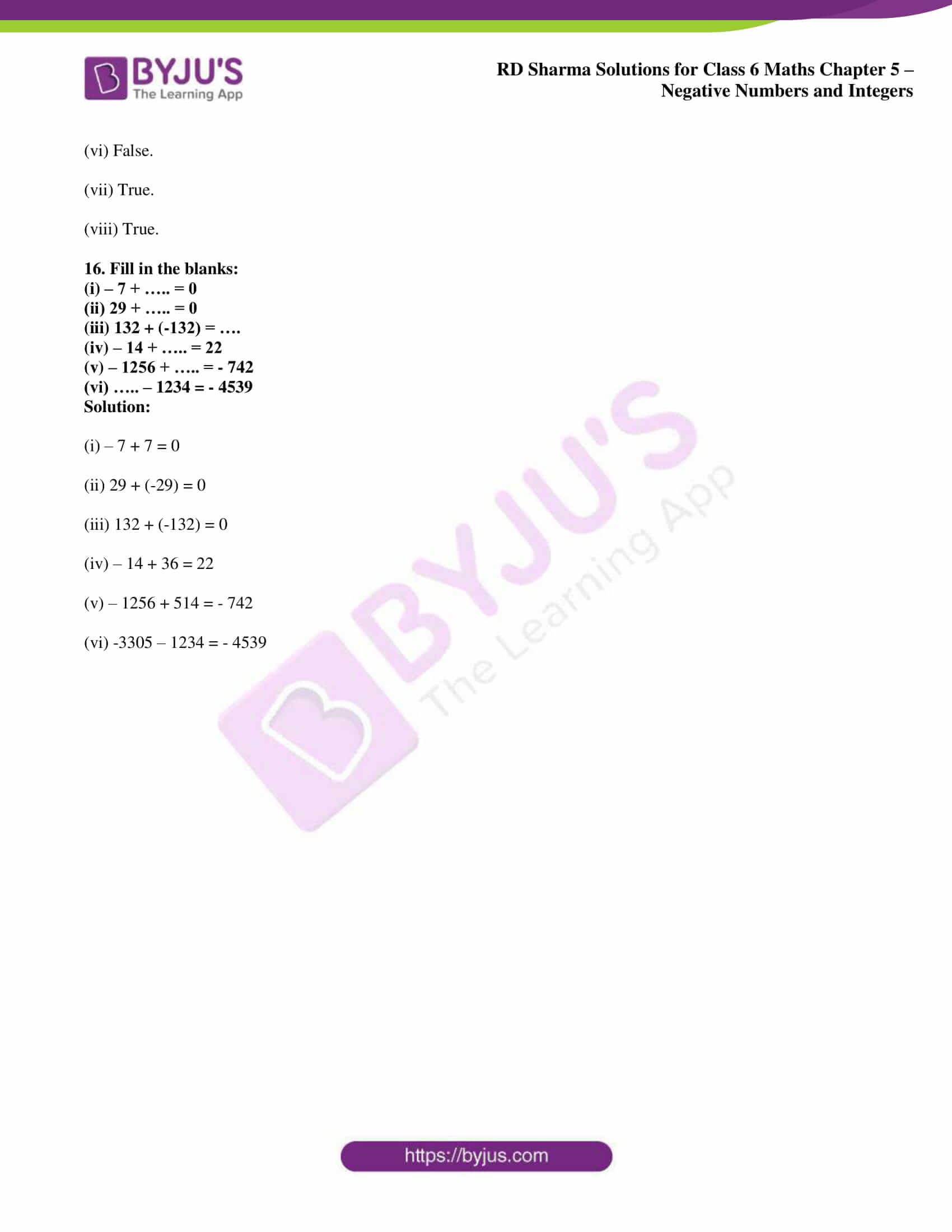## Access answers to Maths RD Sharma Solutions for Class 6 Chapter 5: Negative Numbers and Integers Exercise 5.4

1. Subtract the first integer from the second in each of the following:

(i) 12, -5

(ii) – 12, 8

(iii) – 225, – 135

(iv) 1001, 101

(v) – 812, 3126

(vi) 7560, – 8

(vii) – 3978, – 4109

(viii) 0, – 1005

Solution:

(i) 12, -5

So by subtracting the first integer from the second

-5 – 12 = – 17

(ii) – 12, 8

So by subtracting the first integer from the second

8 – (-12) = 8 + 12 = 20

(iii) – 225, – 135

So by subtracting the first integer from the second

-135 – (-225) = 225 – 135 = 90

(iv) 1001, 101

So by subtracting the first integer from the second

101 – 1001 = – 900

(v) – 812, 3126

So by subtracting the first integer from the second

3126 – (-812) = 3126 + 812 = 3938

(vi) 7560, – 8

So by subtracting the first integer from the second

-8 – 7560 = – 7568

(vii) – 3978, – 4109

So by subtracting the first integer from the second

-4109 – (-3978) = – 4109 + 3978 = -131

(viii) 0, – 1005

So by subtracting the first integer from the second

-1005 – 0 = – 1005

2. Find the value of:

(i) – 27 – (- 23)

(ii) – 17 – 18 – (-35)

(iii) – 12 – (-5) – (-125) + 270

(iv) 373 + (-245) + (-373) + 145 + 3000

(v) 1 + (-475) + (-475) + (-475) + (-475) + 1900

(vi) (-1) + (-304) + 304 + 304 + (-304) + 1

Solution:

(i) – 27 – (- 23)

So we get

= – 27 + 23

On further calculation

= 23 – 27

We get

= – 4

(ii) – 17 – 18 – (-35)

So we get

= – 35 + 35

On further calculation

= 0

(iii) – 12 – (-5) – (-125) + 270

So we get

= – 12 + 5 + 125 + 270

On further calculation

= 400 – 12

We get

= 388

(iv) 373 + (-245) + (-373) + 145 + 3000

So we get

= 373 – 245 – 373 + 145 + 3000

On further calculation

= 3145 + 373 – 373 – 245

We get

= 3145 – 245

By subtraction

= 2900

(v) 1 + (-475) + (-475) + (-475) + (-475) + 1900

So we get

= 1 – 950 – 950 + 1900

On further calculation

= 1900 + 1 – 1900

We get

= 1

(vi) (-1) + (-304) + 304 + 304 + (-304) + 1

So we get

= – 1 + 1 – 304 + 304 – 304 + 304

On further calculation

= 0

3. Subtract the sum of – 5020 and 2320 from – 709.

Solution:

We know that the sum of -5020 and 2320 is

-5020 + 2320

It can be written as

= 2320 – 5020

So we get

= – 2700

Subtracting from – 709 we get

= – 709 – (-2700)

We get

= – 709 + 2700

By subtraction

= 1991

4. Subtract the sum of – 1250 and 1138 from the sum of 1136 and – 1272.

Solution:

We know that the sum of – 1250 and 1138 is

-1250 + 1138

It can be written as

= 1138 – 1250

So we get

= – 112

We know that the sum of 1136 and – 1272 is

1136 – 1272 = – 136

So we get

-136 – (-112) = – 136 + 112 = -24

5. From the sum of 233 and – 147, subtract – 284.

Solution:

We know that the sum of 233 and – 147 is

233 – 147 = 86

Subtracting – 284 we get

86 – (-284) = 86 + 284 = 370

6. The sum of two integers is 238. If one of the integers is – 122, determine the other.

Solution:

It is given that

Sum of two integers = 238

One of the integers = – 122

So the other integer = – (-122) + 238

On further calculation

Other integer = 238 + 122 = 360

7. The sum of two integers is – 223. If one of the integers is 172, find the other.

Solution:

It is given that

Sum of two integers = – 223

One of the integers = 172

So the other integer = – 223 – 172 = – 395

8. Evaluate the following:

(i) – 8 – 24 + 31 – 26 – 28 + 7 + 19 – 18 – 8 + 33

(ii) – 26 – 20 + 33 – (-33) + 21 + 24 – (-25) – 26 – 14 – 34

Solution:

(i) – 8 – 24 + 31 – 26 – 28 + 7 + 19 – 18 – 8 + 33

We get

= – 8 – 24 – 26 – 28 – 18 – 8 + 31 + 7 + 19 + 33

On further calculation

= – 32 – 26 – 28 – 26 + 38 + 19 + 33

It can be written as

= 38 – 32 – 26 – 28 + 33 – 26 + 19

So we get

= 6 – 26 – 28 + 7 + 19

By calculation

= 6 – 28 – 26 + 26

= 6 – 28

By subtraction

= – 22

(ii) – 26 – 20 + 33 – (-33) + 21 + 24 – (-25) – 26 – 14 – 34

We get

= – 46 + 33 + 33 + 21 + 24 + 25 – 26 – 14 – 34

On further calculation

= – 46 + 66 + 21 + 24 + 25 + (-74)

It can be written as

= – 46 + 66 + 70 – 74

So we get

= – 46 – 4 + 66

By calculation

= – 50 + 66

= 66 – 50

By subtraction

= 16

9. Calculate

1 – 2 + 3 – 4 + 5 – 6 + ……… + 15 – 16

Solution:

It can be written as

1 – 2 + 3 – 4 + 5 – 6 + 7 – 8 + 9 – 10 + 11 – 12 + 13 – 14 + 15 – 16

We get

= – 1 – 1 – 1 – 1 – 1 – 1 – 1 – 1

By calculation

= – 8

10. Calculate the sum:

5 + (-5) + 5 + (-5) + …..

(i) if the number of terms is 10.

(ii) if the number of terms is 11.

Solution:

(i) if the number of terms is 10

We get

5 + (-5) + 5 + (-5) + 5 + (-5) + 5 + (-5) + 5 + (-5)

On further calculation

= 5 – 5 + 5 – 5 + 5 – 5 + 5 – 5 + 5 – 5 = 0

(ii) if the number of terms is 11

We get

5 + (-5) + 5 + (-5) + 5 + (-5) + 5 + (-5) + 5 + (-5) + 5

On further calculation

= 5 – 5 + 5 – 5 + 5 – 5 + 5 – 5 + 5 – 5 + 5 = 5

11. Replace * by < or > in each of the following to make the statement true:

(i) (-6) + (-9) * (-6) – (-9)

(ii) (-12) – (-12) * (-12) + (-12)

(iii) (-20) – (-20) * 20 – (65)

(iv) 28 – (-10) * (-16) – (-76)

Solution:

(i) (-6) + (-9) < (-6) – (-9)

(ii) (-12) – (-12) > (-12) + (-12)

(iii) (-20) – (-20) > 20 – (65)

(iv) 28 – (-10) < (-16) – (-76)

12. If △ is an operation on integers such that a △ b = – a + b – (-2) for all integers a, b. Find the value of

(i) 4 △ 3

(ii) (-2) △ (-3)

(iii) 6 △ (-5)

(iv) (-5) △ 6

Solution:

(i) 4 △ 3

By substituting values in a △ b = – a + b – (-2)

We get

4 △ 3 = – 4 + 3 – (-2) = 1

(ii) (-2) △ (-3)

By substituting values in a △ b = – a + b – (-2)

We get

(-2) △ (-3) = – (-2) + (-3) – (-2) = 1

(iii) 6 △ (-5)

By substituting values in a △ b = – a + b – (-2)

We get

6 △ (-5) = – 6 + (-5) – (-2) = – 9

(iv) (-5) △ 6

By substituting values in a △ b = – a + b – (-2)

We get

(-5) △ 6 = – (-5) + 6 – (-2) = 13

13. If a and b are two integers such that a is the predecessor of b. Find the value of a – b.

Solution:

It is given that a is the predecessor of b

We can write it as

a + 1 = b

So we get

a – b = – 1

14. If a and b are two integers such that a is the successor of b. Find the value of a – b.

Solution:

It is given that a is the successor of b

We can write it as

a – 1 = b

So we get

a – b = 1

15. Which of the following statements are true:

(i) – 13 > – 8 – (-2)

(ii) – 4 + (-2) < 2

(iii) The negative of a negative integer is positive.

(iv) If a and b are two integers such that a > b, then a – b is always a positive integer.

(v) The difference of two integers is an integer.

(vi) Additive inverse of a negative integer is negative.

(vii) Additive inverse of a positive integer is negative.

(viii) Additive inverse of a negative integer is positive.

Solution:

(i) False.

(ii) True.

(iii) True.

(iv) True.

(v) True.

(vi) False.

(vii) True.

(viii) True.

16. Fill in the blanks:

(i) – 7 + ….. = 0

(ii) 29 + ….. = 0

(iii) 132 + (-132) = ….

(iv) – 14 + ….. = 22

(v) – 1256 + ….. = – 742

(vi) ….. – 1234 = – 4539

Solution:

(i) – 7 + 7 = 0

(ii) 29 + (-29) = 0

(iii) 132 + (-132) = 0

(iv) – 14 + 36 = 22

(v) – 1256 + 514 = – 742

(vi) -3305 – 1234 = – 4539

### RD Sharma Solutions for Class 6 Maths Chapter 5 – Negative Numbers and Integers Exercise 5.4

RD Sharma Solutions Class 6 Maths Chapter 5 Negative Numbers and Integers Exercise 5.4 provides the students with knowledge about subtraction of integers and various properties of subtraction.

### Key features of RD Sharma Solutions for Class 6 Maths Chapter 5: Negative Numbers and Integers Exercise 5.4

• RD Sharma Solutions help students get an overall understanding about the concepts which are covered.
• The students become more familiar with the problems by solving examples and exercise wise problems from the RD Sharma textbook.
• Good practice of problems mainly boosts confidence among students to perform well in the exam.
• It helps students answer problems more accurately, thus enhancing their problem solving abilities.